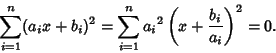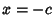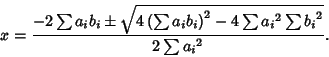## Cauchy Inequality

A special case of the Hölder Sum Inequality with,(1)

where equality holds for. In 2-D, it becomes(2)

It can be proven by writing(3)

Ifis a constant, then. If it is not a constant, then all terms cannot simultaneously vanish for Real, so the solution is Complex and can be found using the Quadratic Equation(4)

In order for this to be Complex, it must be true that(5)

with equality whenis a constant. The Vector derivation is much simpler,(6)

where(7)

and similarly for.